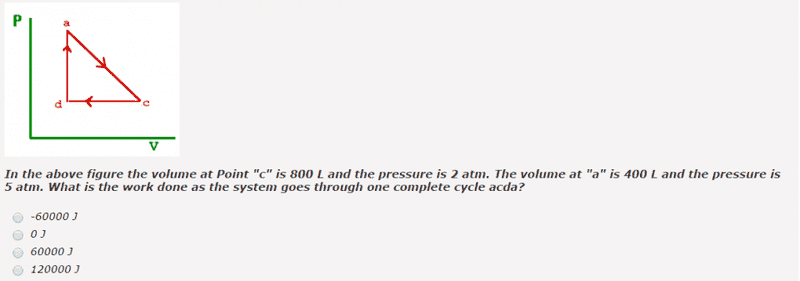# Need Help Solving for Work Done: 60,000 J

• JFS321
L In summary, the question is about finding the correct amount of work done in a gas compression process and the correct answer is 60,000 J according to the book. The conversation discusses various calculations and conversions, ultimately leading to the realization that the conversion factor may be approximated as 1:100. Therefore, the original calculation of work done by the gas on the surroundings is corrected to 3.5 atm and the answer is very close to the expected 60,000 J.

#### JFS321

All, I need some direction on the following question, please.
Correct answer is 60,000 J according to book.

Here's what I have so far, but it may be incorrect: Work done C-->D is (2 atm)(-400 L)(101) = -81,000 J, so work was done on the system when the gas was compressed. No work is done D-->A. Lastly, work done A-->C is (3 atm)(400 L)(101) = 121,200 J. This is work done by the gas on the surroundings. As you can see, my figures do not get to 60,000 J! Thanks in advance. Hopefully I haven't done anything too stupidly obvious!For A-->C, why did you use 3atm? Shouldn't it be the average pressure?

units for pressure should be Pa and units for volume should be m3

The conversion was handled with the (101) term.
1 L-atm = 101.325 J
Perhaps the problem assumes a simpler conversion factor of approx. 1:100.

Yes, it should be 3.5 atm and this gets me very close to the 60,000 J answer. Thank you.

RUber said:
The conversion was handled with the (101) term.
1 L-atm = 101.325 J
Perhaps the problem assumes a simpler conversion factor of approx. 1:100.
RUber said:
The conversion was handled with the (101) term.
1 L-atm = 101.325 J
Perhaps the problem assumes a simpler conversion factor of approx. 1:100.

sorry...I see that the units were taken care of 103 x 10-3